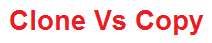Interview Questions

# Difference between Clone and Copy

Clone and Copy ?

Clone will copy the structure of a data where as Copy will copy the complete structure as well as data.Consider this string[] example:

string[] arr1 = new string[] { "one", "two", "three","four" }; string[] arr2 = arr1; //copy

Here declare two string array arr1 and arr2 and then copy arr1 to arr2.

The arr2 now contains the same values, but more importantly the array object itself points to the same object reference as the arr1 array.

Here now assign the value to arr2

arr2 = "zero";

Now display the values in message box, What would be the output for the following statements?

MessageBox.Show(arr1.ToString() + " , " + arr2.ToString());

Considering both arrays point to the same reference we would get:

zero , zero

Form the next sample code you can understand how clone() is working.

string[] arr3 = new string[] { "one", "two", "three", "four" }; string[] arr4 = (string[])arr3.Clone(); //clone

The arr4 now contains the same values, but in this case the array object itself points a different reference than the arr3.

arr4 = "zero";

What would be the output now for the following statements?

MessageBox.Show(arr3.ToString() + " , " + arr4.ToString());
two , zero

Differences between dataset.clone and dataset.copy ?

Both the dataset.Copy and the dataset.Clone methods create a new DataTable with the same structure as the original DataTable. The new DataTable created by the Copy method has the same set of DataRows as the original table, but the new DataTable created by the Clone method does not contain any DataRows

DataTable dt=new DataTable(); dt=ds.Tables.copy();

It will copy all the data and structure to dt table.

DataTable dt=new DataTable(); dt=ds.Table.clone();

It will create only the structure of table not data.